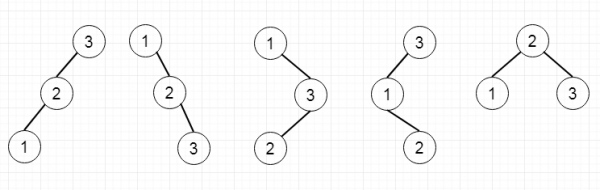# Unique Binary Search Trees II in C++

C++Server Side ProgrammingProgramming

#### C in Depth: The Complete C Programming Guide for Beginners

45 Lectures 4.5 hours

#### Practical C++: Learn C++ Basics Step by Step

Most Popular

50 Lectures 4.5 hours

#### Master C and Embedded C Programming- Learn as you go

66 Lectures 5.5 hours

Suppose we have an integer n, we have to generate all structurally unique binary search trees that store values from 1 to n. So if the input is 3, then the trees will be −To solve this, we will follow these steps −

• Define one recursive function called generate(), this will take low and high
• define one tree node called temp.
• if low > high, then insert null into the temp, and return temp
• for i in range low to high
• left_subtree := generate(low, i – 1)
• right_subtree := generate(i + 1, high)
• current := i
• for j in range 0 to size of the left_subtree
• for k in range 0 to size of the right_subtree
• curr_node := make one tree node with value current
• left of the curr_node := left_subtree[j]
• right of the curr_node := right_subtree[k]
• insert curr_node into the temp
• return temp.
• Initially call the generate() function with values 1 and n to generate all trees.

## Example(C++)

Let us see the following implementation to get a better understanding −

Live Demo

#include <bits/stdc++.h>
using namespace std;
class TreeNode{
public:
int val;
TreeNode *left, *right;
TreeNode(int data){
val = data;
left = right = NULL;
}
};
void insert(TreeNode **root, int val){
queue<TreeNode*> q;
q.push(*root);
while(q.size()){
TreeNode *temp = q.front();
q.pop();
if(!temp->left){
if(val != NULL)
temp->left = new TreeNode(val);
else
temp->left = new TreeNode(0);
return;
}
else{
q.push(temp->left);
}
if(!temp->right){
if(val != NULL)
temp->right = new TreeNode(val);
else
temp->right = new TreeNode(0);
return;
}
else{
q.push(temp->right);
}
}
}
TreeNode *make_tree(vector<int> v){
TreeNode *root = new TreeNode(v);
for(int i = 1; i<v.size(); i++){
insert(&root, v[i]);
}
return root;
}
void tree_level_trav(TreeNode*root){
if (root == NULL) return;
cout << "[";
queue<TreeNode *> q;
TreeNode *curr;
q.push(root);
q.push(NULL);
while (q.size() > 1) {
curr = q.front();
q.pop();
if (curr == NULL){
q.push(NULL);
}
else {
if(curr->left)
q.push(curr->left);
if(curr->right)
q.push(curr->right);
if(curr == NULL || curr->val == 0){
cout << "null" << ", ";
}
else{
cout << curr->val << ", ";
}
}
}
cout << "]"<<endl;
}
class Solution {
public:
vector<TreeNode*> generate(int low, int high) {
vector <TreeNode*> temp;
if(low > high){
temp.push_back(NULL);
return temp;
}
for(int i = low;i<=high;i++){
vector <TreeNode*> leftSubtree = generate(low,i-1);
vector <TreeNode*> rightSubtree = generate(i+1,high);
int current = i;
for(int j = 0;j<leftSubtree.size();j++){
for(int k =0;k<rightSubtree.size();k++){
TreeNode* currentNode = new TreeNode(current);
currentNode->left = leftSubtree[j];
currentNode->right = rightSubtree[k];
temp.push_back(currentNode);
}
}
}
return temp;
}
vector<TreeNode*> generateTrees(int n) {
if(!n){
vector <TreeNode*> r;
return r;
}
return generate(1,n) ;
}
};
main(){
Solution ob;
vector<TreeNode*> v = ob.generateTrees(3);
for(int i = 0; i<v.size(); i++)
tree_level_trav(v[i]);
}

## Input

3

## Output

[1, 2, 3, ]
[1, 3, 2, ]
[2, 1, 3, ]
[3, 1, 2, ]
[3, 2, 1, ]